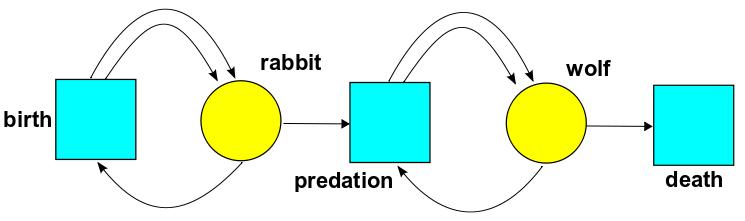# The Azimuth Project Quantum techniques for stochastic mechanics (course) lecture 2 (Rev #2)

## Quantum Techniques for Stochastic Mechanics

• Lecture 2 of 4

• Quantum Techniques for Stochastic Mechanics, by Jacob Biamonte, QIC 890/891 Selected Advanced Topics in Quantum Information, The University of Waterloo, Waterloo Ontario, Canada, (Spring term 2012).

• Given Aug 9th, 2012 in Waterloo Canada

### Lecture Content

• Review of lecture 1

• Probabilities vs amplitudes (chapter 4)

• Operators (creation and annihilation)

• Amoeba field theory

• Preamble to stochastic mechanics vs quantum mechanics

• Note: the lecture content here is to be read along with the youtube video of the course. It should contain what was written on the board. Without the video, it might not make sense.

### The master equation vs the rate equation

A stochastic petri net has

• $k$ species
• $x_i$ is the concetration of the ith species

we want to know

$\frac{d}{d t} x_i = ???$

one for each $1\leq i \leq k$.

• The RHS: sum of terms, one term for each transition in the Petri net

For each transition we end up with a term like this

$\frac{d}{d t} x_i = r (n_i - m_i)x_1^{m_1}\cdots x_k^{m_k}$
• $n_i$ is the number of times species $x_i$ appears in the output
• likewise for $m_i$ but instead for input

We will use index free notation.

$x = (x_1,\ldots, x_k) \in [0, \infinity)$

is the concentration vector and

• input vector: $m = (m_1, \ldots, m_k) \in N^k$

• output vector: $n = (n_1, \ldots, n_k) \in N^k$

• notation: $x^m = x_1^{m_1}\cdots x_k^{m_k}$

then

$\frac{d}{d t} = r(n - m)x^m$
• $T$ is the set of transitions $\tau \in T$
• $r(\tau)$ is the rate constant
$\frac{d}{d t} x = \sum_{\tau \in T} r(\tau) [n(\tau) - m(\tau)]x^m(\tau)$

Example• In birth, one rabbit comes in and two go out. This is a caricature of reality: these bunnies reproduce asexually, splitting in two like amoebas.

• In predation, one wolf and one rabbit come in and two wolves go out. This is a caricature of how predators need to eat prey to reproduce.

• In death, one wolf comes in and nothing goes out. Note that we are pretending rabbits don’t die unless they’re eaten by wolves.

• $R(t)$ rabbits

• $W(t)$ wolves

The rate equations of motion are

$\frac{d}{d t} R(t) = \beta (2-1)R(t) + \gamma (0-1) R(t) W(t)$
$\frac{d} {d t} W(t) = \delta (0-1) W(t) + \gamma (2-1) R(t) W(t)$

### The master equation

• Let $\psi_{n_1, \ldots, n_k} be the probability that we have$ n_1\$